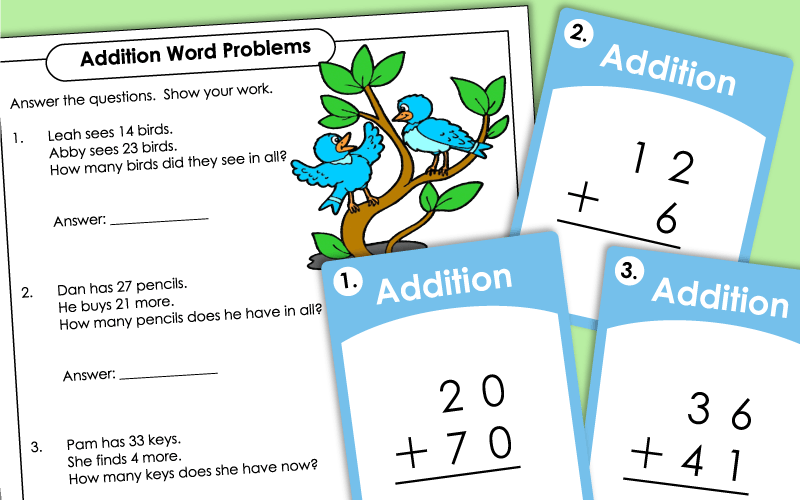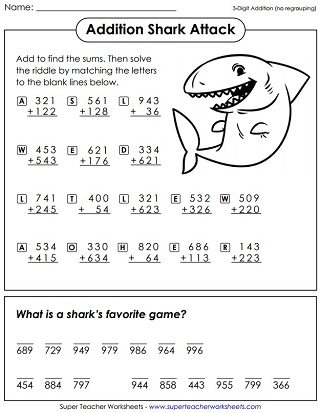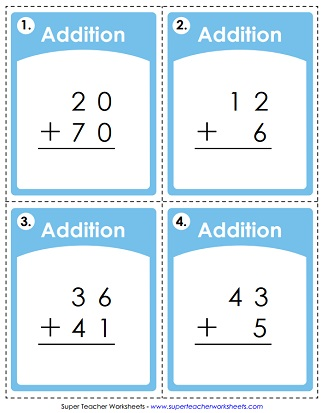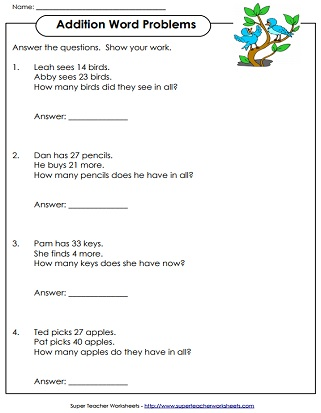# Addition Worksheets (No Regrouping or Borrowing)Solve these addition problems with 2-digit addends. This worksheet does NOT require regrouping.
This worksheet includes 2- and 3-digit addition with no regrouping required.
What's a shark's favorite game? To find out, solve the 3-digit addition problems (no regrouping/carrying). Then use the answers to fill in the answer to the riddle.
Solve these double-digit addition word problems. These problems do NOT require regrouping.
These addition problems are written horizontally. Students rewrite each problem vertically and solve. This worksheet has nine problems.
Simple practice sheet to build addition skills; No carrying the one in these problems!
Add the numbers in the shapes together. For example: Find the sum of the numbers in the trapezoids.
Print out these addition pennants, have each student make a pennant by solving the addition problem, coloring the border, and cutting it out, and glue the pennants along a rope.
Solve the double digit addition facts shown and cut out the cards. Then sort the sums into groups: even and odd. Glue the cards in the correct positions on the t-chart.

This set of 30 task cards has addition problems that do not require carrying. Use these in your learning centers, on your document camera, or for classroom games.
Complete each addition problem by arranging the digits in the correct positions. This game has 12 task cards. Makes a great learning center or small group activity.

Make your own addition-without-regrouping practice pages with our custom worksheet generator tool.

Students will be regrouping (aka borrowing or renaming) numbers on these addition worksheets.

## Sample Worksheet ImagesMy Account
Site Information